Courses

# Test Level 2: Surface Chemistry- 2

## 20 Questions MCQ Test Chemistry Class 12 | Test Level 2: Surface Chemistry- 2

Description
This mock test of Test Level 2: Surface Chemistry- 2 for JEE helps you for every JEE entrance exam. This contains 20 Multiple Choice Questions for JEE Test Level 2: Surface Chemistry- 2 (mcq) to study with solutions a complete question bank. The solved questions answers in this Test Level 2: Surface Chemistry- 2 quiz give you a good mix of easy questions and tough questions. JEE students definitely take this Test Level 2: Surface Chemistry- 2 exercise for a better result in the exam. You can find other Test Level 2: Surface Chemistry- 2 extra questions, long questions & short questions for JEE on EduRev as well by searching above.
QUESTION: 1

### When a graph is plotted between log x/m and log p, it is straight line With an angle 45° and intercept 0.3010 on y-axis. If initial pressure is 0.3 atm, what will be the amount of gas adsorbed per gm of adsorbent :

Solution: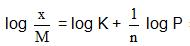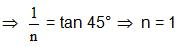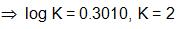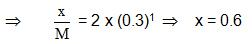QUESTION: 2

### Which of the following statements about physical adsorption is not  correct ?

Solution:

Physical adsorption is a multilayer phenomenon

QUESTION: 3

### Following is the variation of physical adsorption with temperature:

Solution:

QUESTION: 4

Finally divided catalyst has greater surface area and has greater catalytic activity then the compact solid. If a total surface area of 6291456 cm is required for adsorption of gaseous reaction in a catalysed reaction, then how many splits should be made of cube exactly 1 cm in length.

Solution: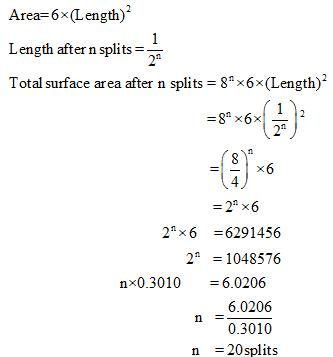QUESTION: 5

Which of the following is not characteristic of chemisorption?

Solution:

Chemical adsorption is a mono layer phenomenon

QUESTION: 6

A colloidal solution can be purified following the method of

Solution:

The Correct answer is option A
A colloidal solution can be purified by the following methods - Dialysis, Electro-dialysis and Ultrafiltration.

QUESTION: 7

Gold number of a lyophilic sol is such property that:

Solution:

Lower is the value gold number; greater will be the protecting power

QUESTION: 8

100 mL of a colloidal solution is completely precipitated by addition of 5 mL of 1 M Nacl solution . Calculate the coagulation value of Nacl .

Solution:

The correct answer is Option C.

Since, coagulation value is defined as the millimoles of an electrolyte that must be added to 1L of solution for coagulation to take place.
No. of millimoles in 5 ml of 1 M NaCl
=  (1/1000) * 5
= 0.005 moles
= 5 moles
No. of millimoles in 100 ml of NaCl
= (5*100)/10
= 50 moles

QUESTION: 9

At CMC, the surfactant molecules :

Solution:

The correct answer is Option C.
In colloidal solution, critical micelle concentration (CMC) is the concentration of surfactants above which micelles form and all additional surfactants added to the system go to micelles, this is because association occurs at CMC.

QUESTION: 10

Some type of gels like gelatin loose water slowly. The process is known as :

Solution:

The correct answer is Option A.

Syneresis is the extraction or expulsion of a liquid from a gel. For example, when lymph drains from a contracting clot of blood.

Thixotropy is a shear thinning property. Certain gels or fluids that are thick (viscous) under static conditions will flow (become thin, less viscous) over time when shaken, agitated or otherwise stressed.

Peptization is the dispersion of a freshly precipitated material into a colloidal solution by action of an electrolyte.

Imbibition is the absorption of a liquid by a solid or gel.

QUESTION: 11

During the adsorption of Krypton on activated charcoal at low temperature

Solution:

During the adsorption of krypton on activated charcoal at low temperature.
ΔH<0 and ΔS<0.
Since adsorption is an exothermic process, the enthalpy change is negative. Heat is given out during the process.
The krypton gas adsorbed on activated charcoal is more ordered than unadsorbed krypton gas. Hence, the process occurs with a decrease in entropy.
In other words, the entropy change is negative for the process.

QUESTION: 12

Size of colloidal particles may range from

Solution:

The correct answer is option A

A colloid is a heterogeneous system in which one substance is dispersed (dispersed phase) as very fine particles in another substance called dispersion medium.
The essential difference between a solution and a colloid is that of particle size. While in a solution, the constituent particles are ions or small molecules, in a colloid, the dispersed phase may consist of particles of a single macromolecule (such as protein or synthetic polymer) or an aggregate of many atoms, ions or molecules.
Colloidal particles are larger than simple molecules but small enough to remain suspended. Their range of diameters is between 1 and 1000 nm

QUESTION: 13

Select correct statement (s) :

Solution:

The correct answer is Option D.
Hydrophilic colloid:- The colloid particles are attracted towards water. They have high viscosity due to strong attraction between the dispersed phase and water.
Hydrophobic colloid: The colloid particles are repelled by water.
They are called irreversible sols. Hydrophobic sols are often formed when a solid crystallizes rapidly from a chemical reaction or a supersaturated solution. When crystallization occurs rapidly, many centers of crystallization (called nuclei) form at the same time. Ions are attracted to these nuclei and very small crystals form. These charged crystals repel one another, so aggregation to larger particles is prevented.
They have a lack of attraction between the dispersed phase and water.

QUESTION: 14

Gold number of haemoglobin is 0.03. Hence, 100 mL of gold sol will require haemoglobin so that gold is not coagulated by 10 mL of 10% NaCl solution:

Solution:

Gold number is the number of milligram of the protective colloid which prevent the coagulation of 10 ml of red gold sol when 1 ml of a 10 percent solution of sodium chloride is added to it.

Here, Gold number of Hb =0.03.

So weight of Hb require for 10 ml of gold is 0.03 mg.

So weight require for 100 ml of gold solution is 0.30mg.

QUESTION: 15

Which one of the following statements is correct:

Solution:

The correct answer is option A

Smaller the size and lesser the viscosity, the faster the motion. Brownian movement arises because of the impact of the molecules of the dispersion medium with the colloidal particles.

As the size of the particle increases, the effect of the impacts average out and the Brownian movement becomes slow. Ultimately, when the dispersed particle becomes big enough to acquire the dimensions of suspension, no Brownian movement is observed.

QUESTION: 16

What can adsorb larger volume of hydrogen gas :

Solution:

It is because of more surface area of colloidal Pd

QUESTION: 17

Statement-1 : All colloidal dispersions give very low osmotic pressure and show very small freezing point depression or boiling pointelevation.

Statement-2 : Tydall effect is due to scattering of light from the surface of colloidal particles.

Solution:

The Tyndall effect is seen due to light scattering by particles in a colloid or particles in a fine suspension. So, solution particles show the Tyndall effect due to the scattering of light and scattering is directly proportional to the size of sol particles.

Also, colloidal particles have a high molar mass so, their mole fraction is very less causing low colligative properties and hence, all colloidal dispersion give very low osmotic pressure and show very small freezing point depression or boiling point elevation.

QUESTION: 18

Statement-1 : The Brownian movement is due to the bombardment of   collodial particles by the molecules of dispersion medium which are in the constant motion like molecules in a gas.

Statement-2 :Brownian movement provides a visible proof of the random kinetic motion of molecules in a liquid.

Solution:

The correct answer is option B

Reason for statement 1 will be the movement is due to the uneven bombardment of the colloidal particles in the dispersion medium.
Statement-1 is True, Statement-2 is True; Statement-2 is NOT a  correct explanation for Statement-1.

QUESTION: 19

Statement-1: In the coagulation of negatively charged arsenic sulphide soil, the coagulating power decreases in the order, Al3+ > Ba2+ > Na+.

Statement-2 : Generally greater the valence of coagulating ion, the  greater is its power of coagulation.

Solution:

The correct answer is option A
Greater the valency of flocculating ions added, the greater its power to cause precipitation.
According to Hardy-Schulz rule, the coagulation (flocculating) value is inversely proportional to the charge on effective ions.
Hence, smaller the value, larger the power of an ion.

QUESTION: 20

Assertion: Isoelectric point is pHpH at which colloidal can move towards either of electrode.
Reason: At isoelectric point coolidal particles becomes electrically netural .

Solution:

At isoelectric point, colloid particles will not move towards either of electrodse because they will come chargeless .